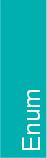## CGAL::vertex_point_t

#include <CGAL/boost/graph/properties.h>

 enum vertex_point_t { vertex_point};

The constant vertex_point is a property tag which refers to the geometric embedding property of a vertex of a HalfedgeGraph.

A vertex_point is an interior property, that is, a property map for a vertex_point can be extracted from any model of a HalfedgeGraph using the BGL PropertyGraph interface:

boost::get(vertex_point,graph)

A point of a vertex can be directly accessed via:

boost::get(vertex_point,graph,vertex)
boost::put(vertex_point,graph,vertex,newpoint)